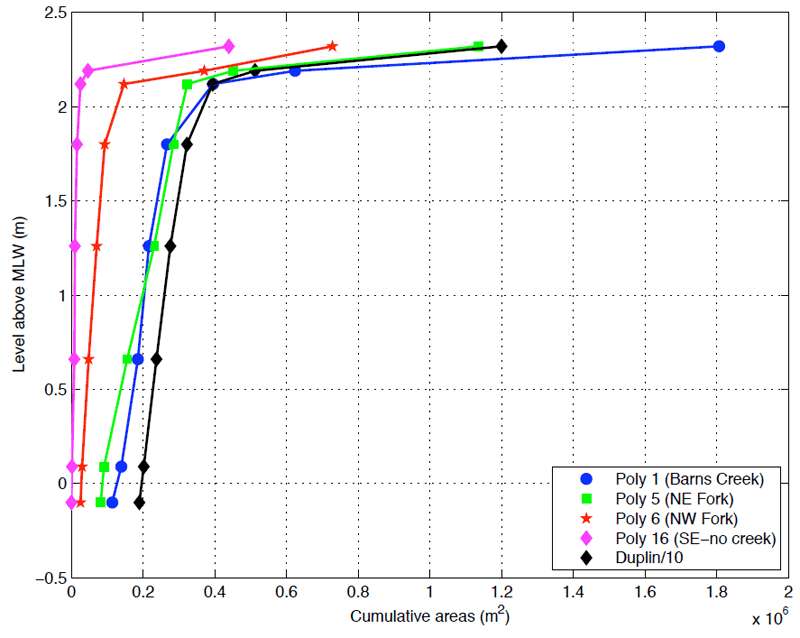# Figure 5: A comparison of hypsometric curves for four polygons with the total drainage area.Figure 5: A comparison of hypsometric curves for four polygons with the total drainage area. The final line-segment in each plot connects water depths and areas from 2.19 to 2.30 meters. The area at 2.19 m references the largest water area calculated for the final overflight (T7). The area at 2.30 m (the water level of the NOAA-published mean spring HHW) references the total polygon area. Areas for the total Duplin tidal water shed have been divided by ten to scale that curve with the polygons.